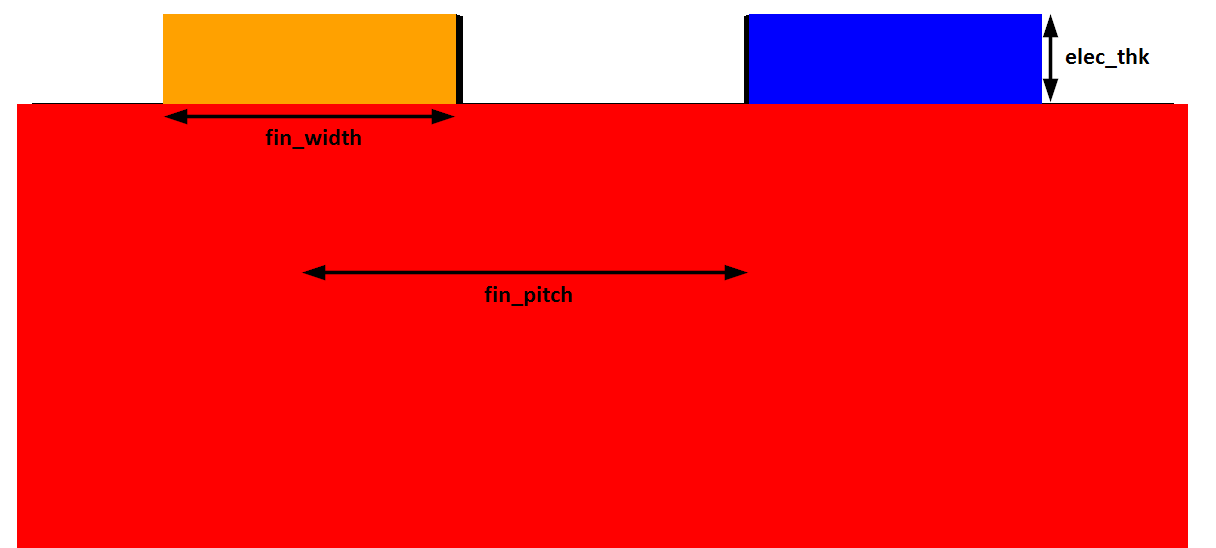# SAW Unit Cell Monte Carlo Study

Monte Carlo Simulation a statistical method which uses simulation to model the probability of outcomes of a complex model whose behaviour cannot be easily determined due to a vast number of variables. Filter performance is affected by a number of design variables.

In this example we vary both the finger pitch, finger width and electrode thickness to get a better idea of the design space and how the key performance (KPIs) relate to these inputs.

## Model Setup

A schematic of the model and the three input variables can be seen below:The key model parameters were as follows. finger pitch, finger width and electrode thickness are set as design variables to be randomly varied (indicated by *).

Design Variable Description Default Value
subs_thk Substrate thickness 7.5 um
elec_thk* Electrode thickness 200 nm
fin_pitch* Finger pitch 1.3 um
aratio Aspect ratio 0.5
fin_width* Finger width 65 um
fin_gap Finger gap 0.65 um
nfing  Number of fingers 100
fin_len Finger Length 50 um
cut_ang Rotated Y-cut angle 42

1000 random input variables were created with the following constraints:

• Finger pitch: 1.3 um ± 12.3%
• Finger width: 65 um ± 0.3%
• Electrode thickness: 200 nm ± 0.3%

## Monte Carlo Results

The full study was completed in 12 minutes when using 2 cores per simulation and had a total cost of 36.28 Core-Hours.

Using the outputs from the simulations, it is possible to calculate the KPIs such as resonant frequencies and Q. The inputs and outputs can then be plot in a number of ways to get insight into the device performance. Results are plotted below in MATLAB.

## Try this Yourself

To run this Monte Carlo study you will need to download the OnScale and MATLAB files.